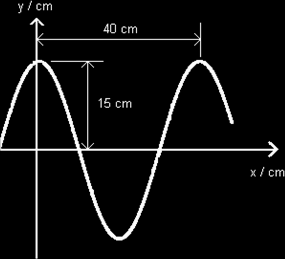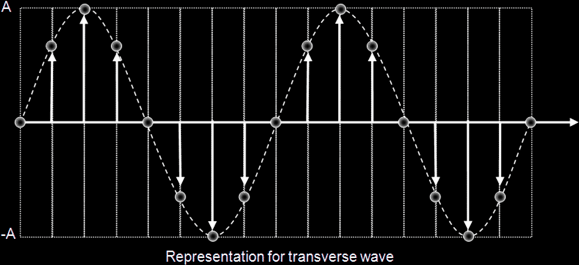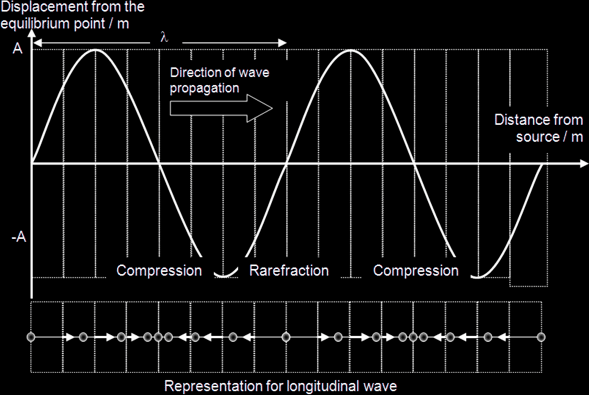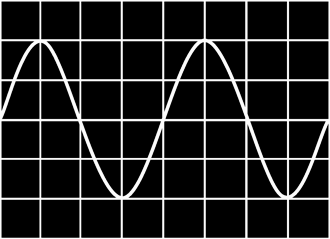# Wave Motion

Displacement (y): Position of an oscillating particle from its equilibrium position.

Amplitude (y0 or A): The maximum magnitude of the displacement of an oscillating particle from its equilibrium position.

Period (T): Time taken for a particle to undergo one complete cycle of oscillation.

Frequency (f): Number of oscillations performed by a particle per unit time.

Wavelength (λ): For a progressive wave, it is the distance between any two successive particles that are in phase, e.g. it is the distance between 2 consecutive crests or 2 troughs.

Wave speed (v): The speed at which the waveform travels in the direction of the propagation of the wave.

Wave front: A line or surface joining points which are at the same state of oscillation, i.e. in phase, e.g. a line joining crest to crest in a wave.

Ray: The path taken by the wave. This is used to indicate the direction of wave propagation. Rays are always at right angles to the wave fronts (i.e. wave fronts are always perpendicular to the direction of propagation).

From the definition of speed, Speed = Distance / Time
A wave travels a distance of one wavelength, λ, in a time interval of one period, T.
The frequency, f, of a wave is equal to 1 / T
Therefore, speed, v = λ / T = (1 / T)λ = fλ

v = fλ

 Example 1: A wave travelling in the positive x direction is showed in the figure. Find the amplitude, wavelength, period, and speed of the wave if it has a frequency of 8.0 Hz. Amplitude (A) = 0.15 m Wavelength (λ) = 0.40 m Period (T) = 1f = 18.0 ≈ 0.125 s Speed (v) =fλ = 8.0 x 0.40 = 3.20 m s-1 A wave which results in a net transfer of energy from one place to another is known as a progressive wave.Intensity {of a wave}: is defined as the rate of energy flow per unit time {power} per unit cross-sectional area perpendicular to the direction of wave propagation.

Intensity = Power / Area = Energy / (Time x Area)

For a point source (which would emit spherical wavefronts),

Intensity = (½mω2xo2) / (t x 4πr2) where x0: amplitude & r: distance from the point source.
Therefore, I ∝ xo2 / r2 (Pt Source)

For all wave sources, I ∝ (Amplitude)2

#### Transverse wave:

A wave in which the oscillations of the wave particles {NOT: movement} are perpendicular to the direction of the propagation of the wave.#### Longitudinal wave:

A wave in which the oscillations of the wave particles are parallel to the direction of the propagation of the wave.Polarisation is said to occur when oscillations are in one direction in a plane, {NOT just “in one direction”} normal to the direction of propagation.

 {Only transverse waves can be polarized; longitudinal waves can’t.} Example 2: The following stationary wave pattern is obtained using a C.R.O. whose screen is graduated in centimetre squares. Given that the time-base is adjusted such that 1 unit on the horizontal axis of the screen corresponds to a time of 1.0 ms, find the period and frequency of the wave. Period, T = (4 units) x 1.0 = 4.0 ms = 4.0 x 10-3 s f = 1 / T = 14 x 10-3 = 250 Hz